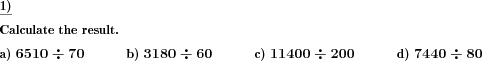Custom math worksheets at your fingertipsDetails for problem "Division by multiples of power of ten"

Quickname: 6894

Elementary School, Primary School.

Summary

Divison by a power of ten or a multiple thereof.

ExampleDescription

Given whole numbers are to be divided by powers of ten or multiples of powers of ten (10,100,1000, etc. or 50,300,2000 etc.).

The number of digits of the power of ten can be specified.

In addition, it can be selected whether only real powers of ten are possible for the divisor or whether it is also possible to divide by a multiple of a power of ten. In the latter case, the multiple can be chosen so that it can come either from the number range 2-9 or from the number range 11-99.

Each problem contains a division task. The number of problems is adjustable.

Download free printable worksheets for this math problem here. The worksheet contains the problems only, the solution sheet includes the answers. Just click on the respective link.

•Worksheet 1Solution sheet with answers
•Worksheet 2Solution sheet with answers
•Worksheet 3Solution sheet with answers

If you can not see the solution sheets for download, they may be filtered out by an ad blocker that you may have installed. If this is the case, please allow ads for this page and reload the page. The solution sheets will then reappear.

• Do these sample worksheets do not really fit?
• Do you need more math worksheets, with a different level of difficulty?
• Would you like to combine different problems on a worksheet and adjust them to your needs?
• As a teacher, you can put together your own worksheets using the automatically generated math problems provided.
With a free initial credit, you can start creating your own math worksheets in a few minutes.

You can try it for free! Register here, to create custom worksheets now!

Customization options for this problem

Parameter
Possible values
Number of problems
1, 2, 3, 4, 5, 6, 7, 8, 9, 10
Powers of ten
10-100, 10-1000, 10-10000, 10-100000
Multiples of p.o.10
no, 2-9, 11-99

Similar problems

Remark
Description
As a division problem with a remainder
Division of two whole numbers, with remainder, written long division method illustrated.Deutsche Version dieser Aufgabe
These informational pages with samples describe math problems that can be combined on custom math worksheets with solutions for home and K-12 school use.
Deutsche Seiten
×